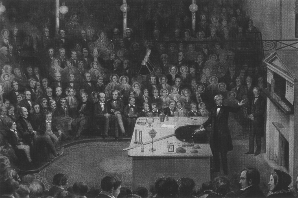# Natural Science - Year II

#### Unit 51: Electricity, Current, and FieldsScience Weblecture for Unit 51

 This Unit's Homework Page History Lecture Science Lecture Lab Parents' Notes

# Science Lecture for Unit 51: Current Electricity

#### For Class

• Topic area: Physics: Electricity
• Terms and concepts to know: Current, resistance, circuit, parallel circuit, series circuit
• See historical period(s): 19th Century

Outline/Summary

## Current Electricity

We've seen how Faraday envisioned a single charged particle influencing the space around it in a kind of "force field", Oersted, Ampère, and Faraday observed the interaction of electrical and magnetic phenomena. All magnetic phenomena is based on moving charges, which then carry the field along. A stationary charge would sense the field of the moving charge as a changing field, becoming stronger as the moving charge nears, and weaker as the moving charge disappears into the distance. All moving charge can be considered current, whether the charge is an electron moving in wire (the most common form), or multiple-atom ions moving through aqueous solutions (as in Volta's electric piles). Again, we need to jump ahead a bit to the model of the atom that the Curies, Thomson, and Rutherford will describe at the beginning of the twentieth century. It isn't Dalton's unbreakable particle, but a complex structure made of positively-charged protons and neutral neutrons in the nucleus, and negatively-charged electrons moving rapidly around the nucleus.

Because of their distance and movement, electrons can become detached from the individual atoms and move through a sample of atoms or molecules, with their speed depending on the composition of this medium. In conducting metals like copper and iron, where atoms are held in a fixed latice structure, electrons can flow freely around the atomic nuclei. In non-conducting substances like carbon, electrons are blocked by the atoms with their own tightly-held electrons. In building circuits where we want electrons to flow, we have to chose materials that will carry the electrons easily.

Moving electrons have kinetic energy, which can be converted through various devices to light (lightbulbs), sound (buzzers and bells) or directed movement (motors). If we connect wires carrying current to such devices, we can use this electrical energy to do "useful work", i.e., light cities, run motors, pump water.

Return to the Learn Physics Today! site for an overview of electrical fields and currents. Read the Electric Current chapter 13 of the Learn Physics Today! site. [1 web page]

• What is current?
• What is resistance?
• How are current and resistance related in a simple circuit?
• Is current conserved in a circuit?

#### Circuits

Now that we understand what current is, and how electrons moving through a wire run into resistance, we can design some paths or circuits for our current to follow. The two main kinds of circuits are series circuits and parallel circuits. In a series circuit, the electrons can follow only one path from their source (the negative battery terminal) through all of the the resistances on the circuit, in order to reach the sink (the positive battery terminal).

Note: By convention, we draw circuits marked with the positive direction of current -- that is, in the direction opposite to the actual flow of the electrons. It's initially confusing, but learning to understand what i → means is easier than determining which convention was used for a particular circuit drawing if we have two conventions.

Read the Basic Circuits, chapter 14 of the Learn Physics Today! site. [1 web page]
• Describe a series circuit with a battery and two resistors.
• Describe a parallel circuit with a battery and two resistors.
• How does the current flow differ in the two circuits?
• What happens if you remove one of the resistors from each circuit?
• How is the energy in a circuit measured?
• How is power in a circuit measured?

### Study/Discussion Questions

• How is your house wired? Where would you expect to find parallel circuits? Why?

### Further Study On your Own (Optional)

• If you are interested in how diodes, LEDs and capacitors work, you can read the final chapter at the Learn Physics Today! site on Advanced Circuits.